# Convective Heat Transfer: One Dimensional (Part - 6) Chemical Engineering Notes | EduRev

## Chemical Engineering : Convective Heat Transfer: One Dimensional (Part - 6) Chemical Engineering Notes | EduRev

The document Convective Heat Transfer: One Dimensional (Part - 6) Chemical Engineering Notes | EduRev is a part of the Chemical Engineering Course Heat Transfer for Engg..
All you need of Chemical Engineering at this link: Chemical Engineering

3.4 Thermal insulation
We have seen how heat transfer is important in various situations. Previous discussion indicates that we are all the time interested in the flow of the heat from one point to another point. However, there are many systems; in fact it is a part of the system, in which we are interested to minimize the losses through heat transfer. For example, in a furnace we want to have high heat transfer inside the furnace; however we do not want any heat loss through the furnace wall. Thus to prevent the heat transfer from the furnace to the atmosphere a bad heat conductor or a very good heat insulator is required. In case of furnace the wall is prepared by multiple layers of refractory materials to minimize the heat losses. Therefore, wall insulation is required in various process equipment, reactors, pipelines etc. to minimize the heat loss from the system to the environment or heat gain from the environment to the system (like cryogenic systems). However, there are situations in which we want to maximize the losses for example, insulation to electric wires.

The petroleum conservation research association (PCRA) provides a good database on the properties and applications of industrial thermal insulations . The table 3.2 shows some common insulations used in chemical process industries for various process equipment and pipelines.

Table-3.2: Thermal properties of a few of the insulations being used in the chemical process industries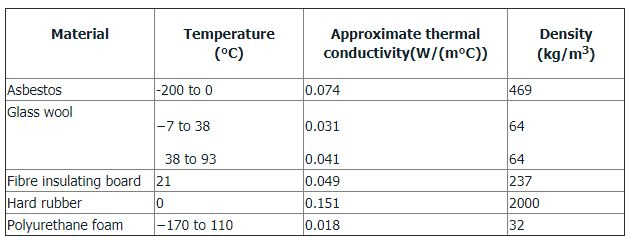An interesting application of the heat loss from a surface of some practical significance is found in the case of insulation of cylindrical surfaces like small pipes or electrical wires. In many a cases we desire to examine the variation in heat loss from the pipe with the change in insulation thickness, assuming that the length of the pipe is fixed. As insulation is added to the pipe, the outer exposed surface temperature will decrease, but at the same time the surface area available to the convective heat dissipation will increase. Therefore, it would be interesting to study these opposing effects.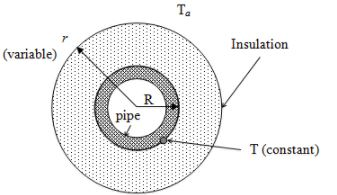Fig. 3.10: Heat dissipation from an insulated pipe

Let us consider a thick insulation layer which is installed around a cylindrical pipe as shown in fig. 3.10 (equivalent electrical circuit is shownin figure 3.11). Let the pipe radius be R and the insulation radius is r. This (r-R) will represent the thickness of the insulation. If the fluid carried by the pipe is at a temperature T and the ambient temperature is Ta. The insulation of the pipe will alter pipe surface temperature T in the radial direction. That is the temperature of the inner surface of the pipe and the outer surface (below insulation) of the pipe will be different. However, if the thermal resistance offered by the pipe is negligible, it can be considered that the temperature (T) is same across the pipe wall thickness and it is a common insulation case (please refer previous discussion). It can also be assumed that the heat transfer coefficient inside the pipe is very high as compared to the heat transfer coefficient at the outside of the insulated pipe. Therefore, only two major resistances in series will be available (insulation layer and gas film of the ambient).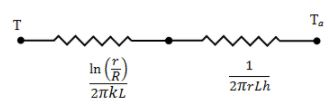Fig.3.11: Resistance offered by the insulation and ambient gas film

Therefore,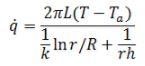where, k is the thermal conductivity of the material.
On differentiating above equation with respect to r will show that the heat dissipation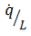reaches a maximum,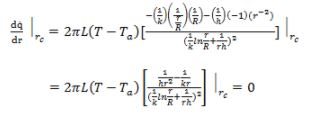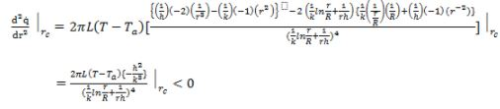So it is maxima, where the insulation radius is equal to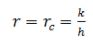where, rc denotes the critical radius of the insulation. The heat dissipation is maximum at rc which is the result of the previously mentioned opposing effects.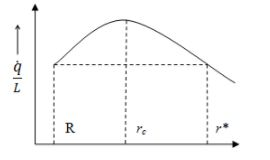Fig. 3.12: The critical insulation thickness of the pipe insulator

Therefore, the heat dissipation from a pipe increases by the addition of the insulation. However, above rc the heat dissipation reduces. The same is shown in fig. 3.12.

The careful analysis of the rc reveals that it is a fixed quantity determined by the thermal properties of the insulator. If R <r, then the initial addition of insulation will increase the heat loss until r =rcand after which it begins to decrease. The same heat dissipation which was at bare pipe radius is again attained at r*. The critical insulation thickness may not always exist for an insulated pipe, if the values of k and h are such that the ratio k/h turns out to be less than R.

It is clear from the above discussion that the insulation above rc reduces the heat dissipation from the cylindrical surface. However, if we keep on increasing the insulation the cost of insulation also increases. Thus again there are two opposing factors that must be considered to obtain the optimum thickness. It should be calculated that what is the pay-back period, that is in how many years the cost of insulation is recovered by the cost of energy saving.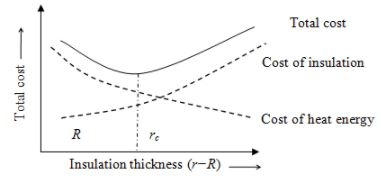Fig. 3.13: Optimum insulation thickness

The optimum insulation thickness (fig. 3.13) can be determined at which the sum of the insulation cost and the cost of the heat loss is minimum.

Offer running on EduRev: Apply code STAYHOME200 to get INR 200 off on our premium plan EduRev Infinity!

## Heat Transfer for Engg.

46 videos|60 docs|59 tests

,

,

,

,

,

,

,

,

,

,

,

,

,

,

,

,

,

,

,

,

,

;